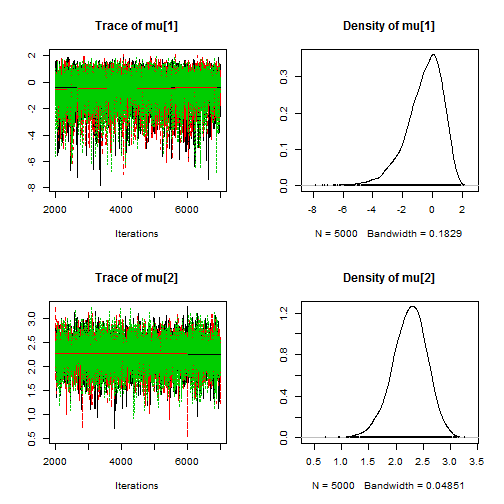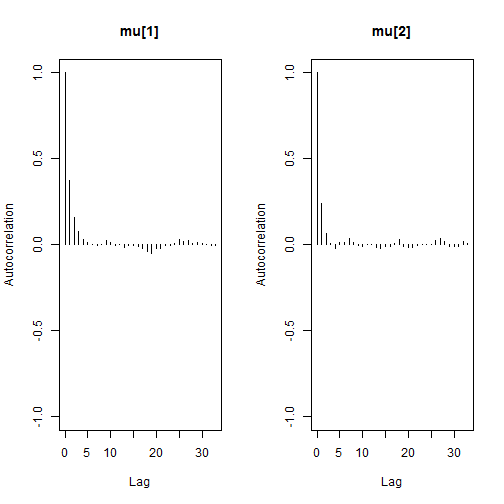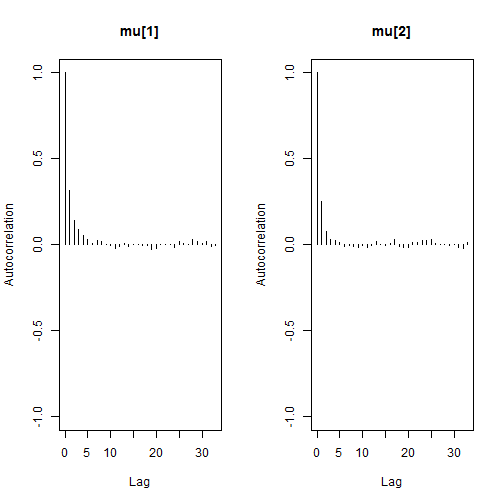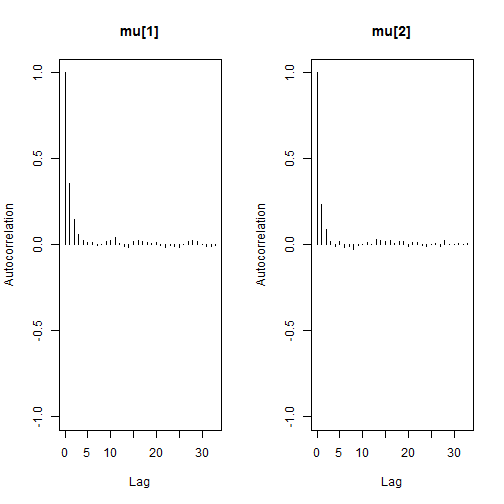# Convergence diagnostics for a well-behaved model

### Chapter 3.4: Diagnosing and improving convergence

In this example the chains do converge and we show how the convergence diagnostics flag convergence. The model is $Y_i\sim\mbox{Poisson}(\exp[\mu_i]) \mbox{ where }\mu_i\sim\mbox{Normal}(0,1000).$ There are two observations: $$Y_1=1$$ and $$Y_2=10$$.

## Define the model as a string

 model_string <- textConnection("model{
Y1    ~ dpois(exp(mu))
Y2    ~ dpois(exp(mu))
mu ~ dnorm(0,0.001)
mu ~ dnorm(0,0.001)
}")


## Generate posterior samples

 inits <- list(mu=rnorm(2,0,5))
data  <- list(Y1=1,Y2=10)
model <- jags.model(model_string,data = data, inits=inits, n.chains=3, quiet=TRUE)

update(model, 1000, progress.bar="none")
samples <- coda.samples(model,
variable.names=c("mu"),
n.iter=5000, progress.bar="none")


## Graphical diagnostics

The trace plots look like bar codes, the chains (each chain is a different color) give similar estimates, and the autocorrelation is near zero for lags 5 and beyond. All of these indicate convergence.

  plot(samples)autocorr.plot(samples)## Numerical diagnostics

  # Low autocorrelation indicates convergence
autocorr(samples[],lag=1)

## , , mu
##
##           mu        mu
## Lag 1 0.3766875 0.0009359125
##
## , , mu
##
##             mu    mu
## Lag 1 -0.02212402 0.241158

  # ESS over 1000 indicates convergence
effectiveSize(samples)

##    mu    mu
## 6622.200 9072.324

  # R less than 1.1 indicates convergence
gelman.diag(samples)

## Potential scale reduction factors:
##
##       Point est. Upper C.I.
## mu          1          1
## mu          1          1
##
## Multivariate psrf
##
## 1

  # |z| less than 2 indicates convergence
geweke.diag(samples[])

##
## Fraction in 1st window = 0.1
## Fraction in 2nd window = 0.5
##
##   mu   mu
## -0.6975 -0.4325